Do you like the article?
Share it with others -

Use new possibilities of MetaTrader 5

# Visualizing optimization results using a selected criterion

31 August 2018, 16:38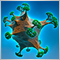0
13 391

### Introduction

We continue developing the application for working with optimization results. Let's consider a case, in which we are able to form the table of the best results after optimizing the parameters by specifying another criterion via the graphical interface. In the previous article, I showed how to work with multiple data categories by saving them in the frame array and extracting them from that array afterwards. This way, we worked with statistical parameters, symbol balance arrays and deposit drawdowns.

After the optimization, we can see the data on separate charts simply by highlighting the corresponding table rows. But there is no limit to perfection. To see the results selected using a certain criterion, it would be good to see their balances all at once on a separate chart. Highlighting rows in the table will form the selected balance curve on this cumulative graph allowing us to better evaluate the optimization result.

Also, at the request of some community members, I will show you how to control highlighting the table rows using the keyboard. To achieve this, I have improved the CTable class in our library.

### Developing the graphical interface

The graphical interface (GUI) of the previous version of our application features three tabs: Frames, Results and Balance.

The Frames tab contains elements for working and viewing all results during and after optimization.

As for the remaining two tabs (Results and Balance), we are going to combine them. Now, we immediately see the result on the graph after highlighting a table row. There is no need to switch to another tab.

The Results tab will feature another group of tabs: Balances and Favorites. The Balances tab contains graphs of multi-symbol balances and depsoit drawdowns, as well as the list of symbols used in the test. The Favorites tab displays the graph of all the best table results. Besides, add the element of the CComboBox type (drop-down list). It will help you choose the criteria for selecting the best results from the general list of frames.

The complete hierarchy of GUI elements now looks like this:

• Form for controls
• Status bar for displaying additional summary information
• The first group of tabs:
• Frames:
• The field for entering the number of displayed result balances when re-scrolling after optimization
• Delay in milliseconds while scrolling results
• Button to start re-scrolling results
• Graph of the specified number of balance results
• Graph displaying all results
• Results:
• Table of best results
• Combo box with a drop-down list for selecting a criterion used to form the table
• The second group of tabs:
• Balance:
• Graph displaying a multi-symbol balance for a selected result
• Graph displaying drawdowns of a selected result
• List of balances for symbols used in the test
• Favorites:
• Graph of the best table results
• Indicator for replaying frames

The code of methods for creating elements is provided in a separate file and included into the file with an MQL program class:

```//+------------------------------------------------------------------+
//| Class for creating the application                               |
//+------------------------------------------------------------------+
class CProgram : public CWndEvents
{
private:
//--- Window
CWindow           m_window1;
//--- Status bar
CStatusBar        m_status_bar;
//--- Tabs
CTabs             m_tabs1;
CTabs             m_tabs2;
//--- Input fields
CTextEdit         m_curves_total;
CTextEdit         m_sleep_ms;
//--- Buttons
//--- Combo boxes
CComboBox         m_criterion;
//--- Graphs
CGraph            m_graph1;
CGraph            m_graph2;
CGraph            m_graph3;
CGraph            m_graph4;
CGraph            m_graph5;
//--- Tables
CTable            m_table_main;
CTable            m_table_symbols;
//--- Progress bar
CProgressBar      m_progress_bar;
//---
public:
//--- Create GUI
bool              CreateGUI(void);
//---
private:
//--- Form
bool              CreateWindow(const string text);
//--- Status bar
bool              CreateStatusBar(const int x_gap,const int y_gap);
//--- Tabs
bool              CreateTabs1(const int x_gap,const int y_gap);
bool              CreateTabs2(const int x_gap,const int y_gap);
//--- Input fields
bool              CreateCurvesTotal(const int x_gap,const int y_gap,const string text);
bool              CreateSleep(const int x_gap,const int y_gap,const string text);
//--- Buttons
bool              CreateReplyFrames(const int x_gap,const int y_gap,const string text);
//--- Combo boxes
bool              CreateCriterion(const int x_gap,const int y_gap,const string text);
//--- Graphs
bool              CreateGraph1(const int x_gap,const int y_gap);
bool              CreateGraph2(const int x_gap,const int y_gap);
bool              CreateGraph3(const int x_gap,const int y_gap);
bool              CreateGraph4(const int x_gap,const int y_gap);
bool              CreateGraph5(const int x_gap,const int y_gap);
//--- Buttons
bool              CreateUpdateGraph(const int x_gap,const int y_gap,const string text);
//--- Tables
bool              CreateMainTable(const int x_gap,const int y_gap);
bool              CreateSymbolsTable(const int x_gap,const int y_gap);
//--- Progress bar
bool              CreateProgressBar(const int x_gap,const int y_gap,const string text);
};
//+------------------------------------------------------------------+
//| Methods for creating controls                                    |
//+------------------------------------------------------------------+
#include "CreateGUI.mqh"
//+------------------------------------------------------------------+```

### Selecting optimization results

To display all the best optimization results on a single chart, we need a method that returns true till the specified number of results is found. Once they are found, the method returns false. This is the CFrameGenerator::UpdateBestResultsGraph() method provided below. 100 best optimization results are displayed by default.

The method applies the double loop. The first loop is limited by the number of displayed best results and the number of table rows to exclude the out of range in the structure of the table data arrays. The frames pointer is moved to the start of the frames list at each iteration of the loop.

In the second loop, we iterate over the frames to find the index of the pass we have previously saved in the arrays structure. The arrays structure should be sorted by the specified criterion before calling the CFrameGenerator::UpdateBestResultsGraph() method. After the pass index is found, get the EA parameters and their number on that pass. After that, get the balance of the current pass result from its data array (m_data[]). Keep in mind that the total balance data is contained in the frame array after the statistical indicators, while the array size is equal to the value in the frame's double parameter. Like the data series, this array is placed on the best results balance graph. If the final result of this test exceeds the initial deposit, the line is green, otherwise — red. The series size is saved in a separate array so that after the end of the loop, it is possible to define a series with the maximum number of elements for setting the X axis boundaries. Finally, the frame counter should be increased by one, so that next time it is possible to continue the loop, while excluding this pass.

If the loop is fully passed:

• the frame counter is reset,
• a series of data with the maximum number of elements is defined,
• parameters are set for the best results graph and it is updated.

After that, the CFrameGenerator::UpdateBestResultsGraph() method returns false meaning that results selection is over.

```//+------------------------------------------------------------------+
//| Class for working with optimization results                      |
//+------------------------------------------------------------------+
class CFrameGenerator
{
private:
//--- Number of best results
int               m_best_results_total;
//---
public:
//--- Update the best results graph
bool              UpdateBestResultsGraph(void);
};
//+------------------------------------------------------------------+
//| Constructor                                                      |
//+------------------------------------------------------------------+
CFrameGenerator::CFrameGenerator(void) : m_best_results_total(100)
{
}
//+------------------------------------------------------------------+
//| Update the best results graph                                    |
//+------------------------------------------------------------------+
bool CFrameGenerator::UpdateBestResultsGraph(void)
{
for(int i=(int)m_frames_counter; i<m_best_results_total && i<m_rows_total; i++)
{
//--- Move the frame pointer to the beginning
::FrameFirst();
//--- Data extraction
while(::FrameNext(m_pass,m_name,m_id,m_value,m_data))
{
//--- Pass index numbers do not match, move to the next one
if(m_pass!=(ulong)m_columns.m_rows[i])
continue;
//--- Get the parameters and their number
GetParametersTotal();
//--- Get the current result balance
double serie[];
::ArrayCopy(serie,m_data,0,STAT_TOTAL,(int)m_value);
//--- Send the array for displaying on the balance graph
CCurve *curve=m_graph_best.CurveGetByIndex(i);
curve.Name((string)m_pass);
curve.Color((m_data[m_profit_index]>=0)? ::ColorToARGB(clrLimeGreen) : ::ColorToARGB(clrRed));
curve.Update(serie);
//--- Get the series size
m_curve_max[i]=::ArraySize(serie);
//--- Increase the frames counter
m_frames_counter++;
return(true);
}
}
//--- Reset the frames counter
m_frames_counter=0;
//--- Define the row with the maximum number of elements
double x_max=m_curve_max[::ArrayMaximum(m_curve_max)];
//--- Horizontal axis properties
CAxis *x_axis=m_graph_best.XAxis();
x_axis.Min(0);
x_axis.Max(x_max);
x_axis.DefaultStep((int)(x_max/8.0));
//--- Update the graph
m_graph_best.CalculateMaxMinValues();
m_graph_best.CurvePlotAll();
m_graph_best.Update();
return(false);
}```

To find the results, go through all the frames in the general list. This takes time. Therefore, use the "Progress bar" element (CProgressBar) to track the search stage. The application class (CProgram) features the CProgram::GetBestOptimizationResults() method for that. Here, in the while loop, the CFrameGenerator::UpdateBestResultsGraph() method is called as a condition. Before starting the loop, make the progress bar visible. Since the frames counter is used in the CFrameGenerator::UpdateBestResultsGraph() method, we can receive its current value. After completing the loop, the progress bar should be hidden.

```class CProgram : public CWndEvents
{
private:
void              GetBestOptimizationResults(void);
};
//+------------------------------------------------------------------+
//+------------------------------------------------------------------+
void CProgram::GetBestOptimizationResults(void)
{
//--- Show the progress bar
m_progress_bar.Show();
//--- Visualize getting the best results
int best_results_total=m_frame_gen.BestResultsTotal();
while(m_frame_gen.UpdateBestResultsGraph() && !::IsStopped())
{
//--- Update the progress bar
m_progress_bar.LabelText("Selection of results: "+string(m_frame_gen.CurrentFrame())+"/"+string(best_results_total));
m_progress_bar.Update((int)m_frame_gen.CurrentFrame(),best_results_total);
}
//--- Hide the progress bar
m_progress_bar.Hide();
}```

The CProgram::GetBestOptimizationResults() method should be called in the optimization completion method. Thus, users will understand that the program is active. Other methods have been considered in the previous articles, so there is no point in dwelling on them.

```//+------------------------------------------------------------------+
//| Optimization completion event                                    |
//+------------------------------------------------------------------+
void CProgram::OnTesterDeinitEvent(void)
{
//--- Optimization completion
m_frame_gen.OnTesterDeinitEvent();
//--- Visualize receiving the best results
GetBestOptimizationResults();
//--- Make the interface available
IsLockedGUI(true);
//--- Calculate the ratio of positive and negative results
CalculateProfitsAndLosses();
//--- Get data to the optimization results table
GetFrameDataToTable();
//--- Initialize the GUI core
CWndEvents::InitializeCore();
}```

Immediately after the optimization completion or its forced stop, the progress bar appears in the status bar. It shows the user that the result selection is in progress: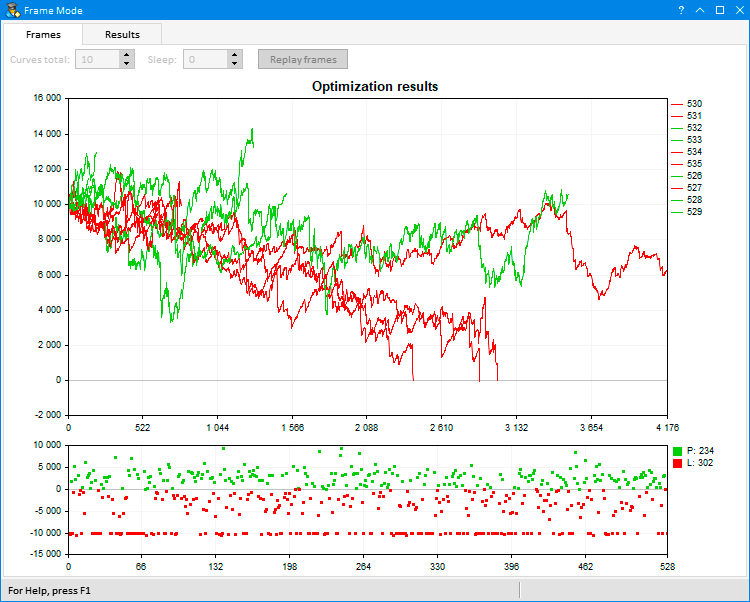Fig. 1. Visualizing results selection

To see the visualization of all selected results, go to the Results tab and then select the Favorites tab. By default, 100 best results by the Profit criterion are added to the table. Another criterion for selecting 100 best results can be selected from the Criterion drop-down list at any moment. We will return to this later. Now let's consider the methods of organizing this process.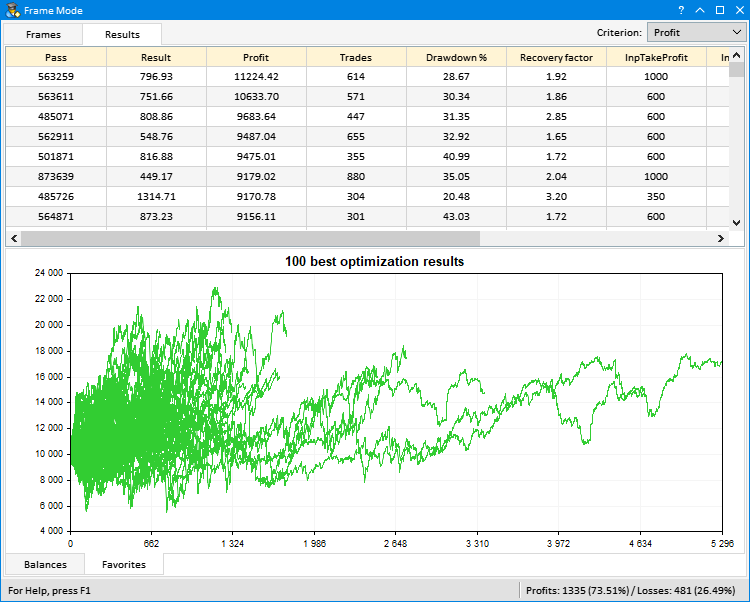Fig. 2. Graph of the best optimization results

### Highlighting a table row programmatically

Until now, we could highlight a table row only by left-clicking on it. But sometimes it needs to be done programmatically — for example, by Up, Down, Home and End keys. To programmatically select a row, the CTable class features the CTable::SelectRow() public method. Its code is similar to the CTable::RedrawRow() private method. In turn, this method is used for redrawing the rows by mouse click events and hovering the cursor over the table when the rows highlighting mode is on.

Much of the code can be re-used in both methods. Therefore, I put it into the separate CTable::DrawRow() method. The things to be passed to it:

• array of indices for defining the redrawing sequence,
• highlighted row's current index,
• previous highlighted row's index,
• method application mode — programmatic (false) or custom (true).
The method itself defines the coordinates for redrawing rows and their elements are drawn in sequence: background, grid, images and text.
```//+------------------------------------------------------------------+
//| Draw the specified table row by the specified mode               |
//+------------------------------------------------------------------+
void CTable::DrawRow(int &indexes[],const int item_index,const int prev_item_index,const bool is_user=true)
{
int x1=0,x2=m_table_x_size-2;
int y1={0},y2={0};
//--- Number of rows and columns for drawing
uint rows_total    =0;
uint columns_total =m_columns_total-1;
//--- If the row highlight method is programmatic
if(!is_user)
rows_total=(prev_item_index!=WRONG_VALUE && item_index!=prev_item_index)? 2 : 1;
else
rows_total=(item_index!=WRONG_VALUE && prev_item_index!=WRONG_VALUE && item_index!=prev_item_index)? 2 : 1;
//--- Draw the rows background
for(uint r=0; r<rows_total; r++)
{
//--- Calculate the coordinates of the row's upper and lower borders
y1[r] =m_rows[indexes[r]].m_y+1;
y2[r] =m_rows[indexes[r]].m_y2-1;
//--- Define the focus on the row relative to the highlighting mode
bool is_item_focus=false;
if(!m_lights_hover)
is_item_focus=(indexes[r]==item_index && item_index!=WRONG_VALUE);
else
is_item_focus=(item_index==WRONG_VALUE)?(indexes[r]==prev_item_index) :(indexes[r]==item_index);
//--- Draw the row background
m_table.FillRectangle(x1,y1[r],x2,y2[r],RowColorCurrent(indexes[r],(is_user)? is_item_focus : false));
}
//--- Draw the borders
for(uint r=0; r<rows_total; r++)
{
for(uint c=0; c<columns_total; c++)
m_table.Line(m_columns[c].m_x2,y1[r],m_columns[c].m_x2,y2[r],::ColorToARGB(m_grid_color));
}
//--- Draw the images
for(uint r=0; r<rows_total; r++)
{
for(uint c=0; c<m_columns_total; c++)
{
//--- Draw the image if (1) it is present in this cell and (2) the text is left-aligned in this column
if(ImagesTotal(c,indexes[r])>0 && m_columns[c].m_text_align==ALIGN_LEFT)
CTable::DrawImage(c,indexes[r]);
}
}
//--- For calculating the coordinates
int x=0,y=0;
//--- text alignment mode
uint text_align=0;
//--- Draw the text
for(uint c=0; c<m_columns_total; c++)
{
//--- Get the text's (1) X coordinate and (2) alignment mode
x          =TextX(c);
text_align =TextAlign(c,TA_TOP);
//---
for(uint r=0; r<rows_total; r++)
{
//--- (1) Calculate the coordinate and (2) draw the text
y=m_rows[indexes[r]].m_y+m_label_y_gap;
m_table.TextOut(x,y,m_columns[c].m_rows[indexes[r]].m_short_text,TextColor(c,indexes[r]),text_align);
}
}
}```

Only one argument — the index of the row to be highlighted — should be passed to the CTable::SelectRow() method. Here, we should first check for the table out of range and if the row with the same index has already been highlighted. Next, define the current and previous indices of the highlighted row and redrawing sequence. Pass the obtained values to the CTable::DrawRow() method. After receiving the indices on the borders of the table's visible area, we should define where to move the scrollbar slider.

```//+------------------------------------------------------------------+
//| Highlight the specified row in the table                         |
//+------------------------------------------------------------------+
void CTable::SelectRow(const int row_index)
{
//--- Check for out of range
if(!CheckOutOfRange(0,(uint)row_index))
return;
//--- If the row already highlighted
if(m_selected_item==row_index)
return;
//--- Rows' current and previous indices
m_prev_selected_item =(m_selected_item==WRONG_VALUE)? row_index : m_selected_item;
m_selected_item      =row_index;
//--- Array for values in a certain sequence
int indexes;
//--- If here for the first time
if(m_prev_selected_item==WRONG_VALUE)
indexes=m_selected_item;
else
{
indexes =(m_selected_item>m_prev_selected_item)? m_prev_selected_item : m_selected_item;
indexes =(m_selected_item>m_prev_selected_item)? m_selected_item : m_prev_selected_item;
}
//--- Draw the specified table row according to the set mode
DrawRow(indexes,m_selected_item,m_prev_selected_item,false);
//--- Get indices on the visible area borders
VisibleTableIndexes();
//--- Move the scroll bar for the specified row
if(row_index==0)
{
VerticalScrolling(0);
}
else if((uint)row_index>=m_rows_total-1)
{
VerticalScrolling(WRONG_VALUE);
}
else if(row_index<(int)m_visible_table_from_index)
{
VerticalScrolling(m_scrollv.CurrentPos()-1);
}
else if(row_index>=(int)m_visible_table_to_index-1)
{
VerticalScrolling(m_scrollv.CurrentPos()+1);
}
}```

The updated version of the CTable class can be downloaded below. The newest version of the EasyAndFast library is available in CodeBase.

### Auxiliary methods of working with frame data

In the application version provided in the previous article, multi-symbol balances and deposit drawdowns are displayed on graphs when highlighting a row in the results table. To understand which symbol a particular curve belongs to on a multisymbol graph, the names of the curves were displayed separately on the right side of the chart. In the current version, the graph size by Y axis is fixed. In case of multiple test symbols, it is impossible to fit them all in the selected area. Therefore, locate the CTable type list with a scroll bar to the right of the graphs. The list will contain all balance names.

The CProgram::GetFrameSymbolsToTable() method is used to receive symbols. After receiving the frame data, it is possible to get result symbols from the string parameter. Get the symbol list after passing a string array. If there are more than one symbols, the number of balances should be increased by one element reserving the first one for the total balance.

Next, set the table size. Here we need only one column, while the number of rows is equal to the number of curves on the graph. Specify the column width and set the table header name. Fill the table with balance names in the loop. To understand, which curve relates to a particular name, we associate them using a color component. Update the elements to display the newly made changes.

```//+------------------------------------------------------------------+
//| Get frame symbols to the table                                   |
//+------------------------------------------------------------------+
void CProgram::GetFrameSymbolsToTable(void)
{
//--- Get the list of symbols and the number of curves
string symbols[];
int symbols_total  =m_frame_gen.CopySymbols(symbols);
int balances_total =(symbols_total>1)? symbols_total+1 : symbols_total;
//--- Set the table size
m_table_symbols.Rebuilding(1,balances_total,true);
//--- List column width
int width[]={111};
m_table_symbols.ColumnsWidth(width);
//--- Fill the table with data from frames
for(uint r=0; r<m_table_symbols.RowsTotal(); r++)
{
uint clr=m_graph3.GetGraphicPointer().CurveGetByIndex(r).Color();
m_table_symbols.TextColor(0,r,::ColorToARGB(clr));
m_table_symbols.SetValue(0,r,(symbols_total>1)?(r<1)? "BALANCE" : symbols[r-1]: symbols[r],0);
}
//--- Update the table
m_table_symbols.Update(true);
m_table_symbols.GetScrollHPointer().Update(true);
m_table_symbols.GetScrollVPointer().Update(true);
}```

It would be very convenient if an appropriate curve was highlighted on the graph when highlighting a result in the table. To achieve this, write the CProgram::SelectCurve() method. it receives an index of a pass for searching the necessary graph curve. Curve names correspond to pass indices they belong to. Therefore, they can be detected simply by comparing the passed index with the one containing in the curve name in a loop. Once the necessary curve is found, remember its index and complete the loop.

Now, the curve should be moved to the upper layer. If we mark the curve by simply changing its color, it may get lost among the others. Instead, we need to swap the found curve and the one that was drawn last.

To do this, get the pointers of these two curves by indices. Then copy their names and data sets. After that, swap them. Set the line width and color for the last curve. Let's make it black, so that it remains visible among the others. Update the graph for the changes to take effect.

```//+------------------------------------------------------------------+
//| Optimization end event                                           |
//+------------------------------------------------------------------+
void CProgram::SelectCurve(const ulong pass)
{
CGraphic *graph=m_graph5.GetGraphicPointer();
//--- Search for the curve by the pass index
ulong curve_index =0;
int curves_total  =graph.CurvesTotal();
for(int i=0; i<curves_total; i++)
{
if(pass==(ulong)graph.CurveGetByIndex(i).Name())
{
curve_index=i;
break;
}
}
//--- Highlighted and last curves on the chart
CCurve *selected_curve =graph.CurveGetByIndex((int)curve_index);
CCurve *last_curve     =graph.CurveGetByIndex((int)curves_total-1);
//--- Copy highlighted and last data array
double y1[],y2[];
string name1=selected_curve.Name();
string name2=last_curve.Name();
selected_curve.GetY(y1);
last_curve.GetY(y2);
//---
last_curve.Name(name1);
selected_curve.Name(name2);
last_curve.Update(y1);
selected_curve.Update(y2);
//---
last_curve.LinesWidth(2);
last_curve.Color(clrBlack);
//--- Update the graph
graph.CurvePlotAll();
graph.Update();
}```

Now, when we highlight a table row, we will also see the appropriate balance curve on the graph.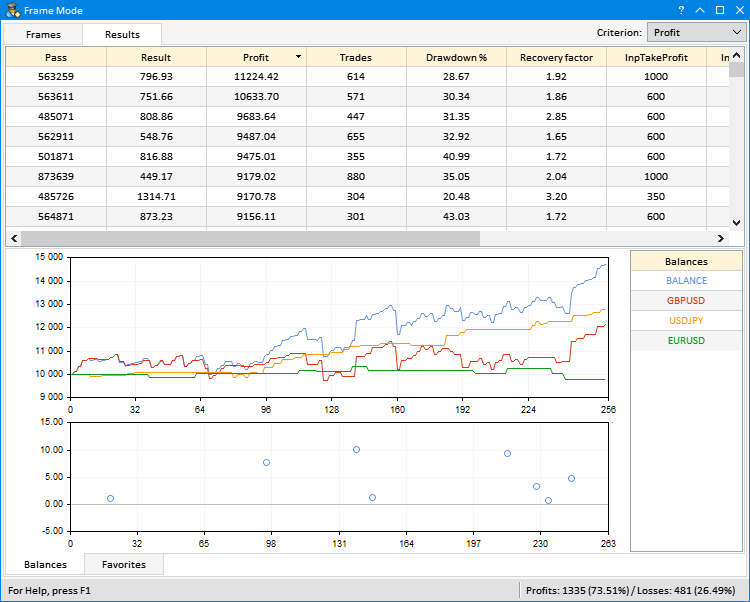Fig. 3. Highlighting the curves on the graph

### Handling events when interacting with the graphical interface

Now, let's consider the event handling methods generated when interacting with the graphical interface of our application. Here are the methods.

• Highlighting a table row by left-clicking on it.
• Selecting results in the table from keyboard.
• Choosing a criterion for the results selection in the drop-down list.

When highlighting a row by clicking on it, the ON_CLICK_LIST_ITEM custom event is generated. The CProgram::TableRowSelection() method is called to handle it. The long parameter of the event is passed to it. This parameter is an ID of the element the event was generated from. If the ID is not related to the element, the program exits the method and checks the next element in the handler of the application element events. If the ID matches the result table's one, we get the index of the pass from the first table column. Therefore, to get the pass number, you just need to specify the column and the newly selected row indices by passing these values ​​to the CTable :: GetValue() method.

So, we got the pass index. Now, we can extract data from this frame followed by symbols contained in this result. Add them to the table on the first tab of the second group. In the end, highlight the balance curve on the graph of all results.

```//+------------------------------------------------------------------+
//| Highlighting the table row by left-clicking on it                |
//+------------------------------------------------------------------+
bool CProgram::TableRowSelection(const long element_id)
{
//--- Highlight the table row
if(element_id!=m_table_main.Id())
return(false);
//--- Get the pass index from the table
ulong pass=(ulong)m_table_main.GetValue(0,m_table_main.SelectedItem());
//--- Get data by the pass index
m_frame_gen.GetFrameData(pass);
//--- Add symbols to the table
GetFrameSymbolsToTable();
//--- Highlight the curve on the graph by the pass index
SelectCurve(pass);
return(true);
}```

As soon as the ON_CLICK_LIST_ITEM custom event arrives, choosing the criterion for results selection in the combo box (CComboBox) drop-down list is handled as well. This is done by the CProgram::ShowResultsBySelectedCriteria() method. After a successful check of the ID element, get the index of a selected item in the drop-down list. In this application version, three criteria are available in the drop-down list:

• Result — custom result returned by the OnTester() function.
• Profit —  total profit of a test result.
• Recovery factor.

Next, define the index of the column with data related to a selected criterion. The first item relates to the column with index 1, the second one — to the column with index 2, the third one — to the column with index 5. Next, get the frames with the best results according to a selected criterion. To do this, call the CFrameGenerator::OnChangedSelectionCriteria() method by passing the column index to it. Now, everything is ready for receiving balances of the best results on the graph. The progress bar visualizes the process. The last call here is receiving all data in the best results table.

```//+------------------------------------------------------------------+
//| Display results by a specified criterion                         |
//+------------------------------------------------------------------+
bool CProgram::ShowResultsBySelectedCriteria(const long element_id)
{
//--- Check the element ID
if(element_id!=m_criterion.Id())
return(false);
//--- Define the criterion index for receiving the best results
int index=m_criterion.GetListViewPointer().SelectedItemIndex();
int column_index=(index<1)? 1 : (index==1)? 2 : 5;
m_frame_gen.OnChangedSelectionCriteria(column_index);
//--- Visualize receiving the best results
GetBestOptimizationResults();
//--- Get data to the optimization results table
GetFrameDataToTable();
return(true);
}```

In the program's even handler, the methods described above are called sequentially upon the ON_CLICK_LIST_ITEM event arrival, until one of them returns true.

```//+------------------------------------------------------------------+
//| Event handler                                                    |
//+------------------------------------------------------------------+
void CProgram::OnEvent(const int id,const long &lparam,const double &dparam,const string &sparam)
{
...
//--- Event of clicking on the table rows
if(id==CHARTEVENT_CUSTOM+ON_CLICK_LIST_ITEM)
{
//--- Highlighting the table rows
if(TableRowSelection(lparam))
return;
//--- Choose a criterion for selecting results
if(ShowResultsBySelectedCriteria(lparam))
return;
//---
return;
}
...
}```

The image below shows selection from the drop-down list, receiving data and their drawing on the graph: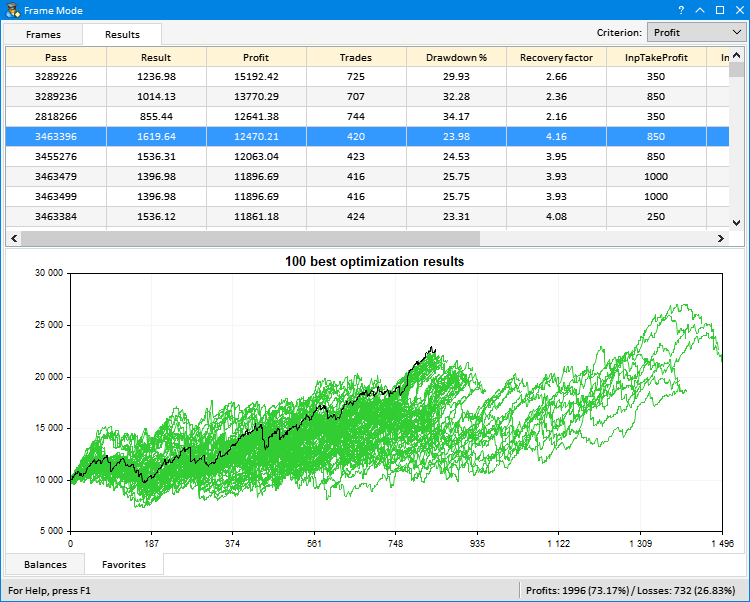Fig. 4. Selecting results by the specified criterion

To select a row from keyboard, the CProgram::SelectingResultsUsingKeys() is required. The code of a pressed button should be passed to it. It arrives in the 'long' parameter of the CHARTEVENT_KEYDOWN event. Get the index of the currently highlighted row in the table at the start of the method. Next, define the pressed button in the 'switch' operator. Below is an example of handling four buttons:

• KEY_UP — if the Up key is pressed, the current index of the highlighted row is decreased by 1. The next upper row is highlighted.
• KEY_DOWN — if the Down key is pressed, the current index of the highlighted row is increased by 1. The next lower row is highlighted.
• KEY_HOME — if the Home key is pressed, set the index of the first table row.
• KEY_END — if the End key is pressed, set the index of the last table row.

Now, it is time to pass the checks. The program exits the method in the following cases:

• if the row is not highlighted,
• if the same row is highlighted,
• if we exit out of the list.

If the checks are passed, the specified row is highlighted in the table and the vertical scroll bar is shifted if necessary.

After highlighting the row, the following happens.

1. Get the pass index from the first table column.
2. Get the data by the pass index.
3. Add symbols to the list next to the multi-symbol graph.
4. In the end, highlight the balance curve on the graph of all selected results.

The CProgram::SelectingResultsUsingKeys() method code:

```//+------------------------------------------------------------------+
//| Select results using keys                                        |
//+------------------------------------------------------------------+
bool CProgram::SelectingResultsUsingKeys(const long key)
{
//--- Get highlighted row index
int selected_row=m_table_main.SelectedItem();
//--- Define direction and row for moving the scroll bar
switch((int)key)
{
case KEY_UP :
selected_row--;
break;
case KEY_DOWN :
selected_row++;
break;
case KEY_HOME :
selected_row=0;
break;
case KEY_END :
selected_row=(int)m_table_main.RowsTotal()-1;
break;
}
//--- Exit if (1) the row is not highlighted or (2) the same previously selected row is highlighted or (3) we exit the list range
if(selected_row==WRONG_VALUE || selected_row==m_table_main.SelectedItem() ||
selected_row<0 || selected_row>=(int)m_table_main.RowsTotal())
return(false);
//--- Highlight the row and shift the scroll bar
m_table_main.SelectRow(selected_row);
m_table_main.Update();
m_table_main.GetScrollVPointer().Update(true);
//--- Get the pass index from the highlighted table row
ulong pass=(ulong)m_table_main.GetValue(0,m_table_main.SelectedItem());
//--- Get data by the pass index
m_frame_gen.GetFrameData(pass);
//--- Add symbols to the table
GetFrameSymbolsToTable();
//--- Highlight the curve on the graph by the pass index
SelectCurve(pass);
return(true);
}```

The CProgram::SelectingResultsUsingKeys() method is called upon arrival of the keyboard pressing event (CHARTEVENT_KEYDOWN) in the program event handler:

```//+------------------------------------------------------------------+
//| Event handler                                                    |
//+------------------------------------------------------------------+
void CProgram::OnEvent(const int id,const long &lparam,const double &dparam,const string &sparam)
{
//--- Pressing the button
if(id==CHARTEVENT_KEYDOWN)
{
//--- Select results using keys
if(SelectingResultsUsingKeys(lparam))
return;
//---
return;
}
...
}```

Here is how it works: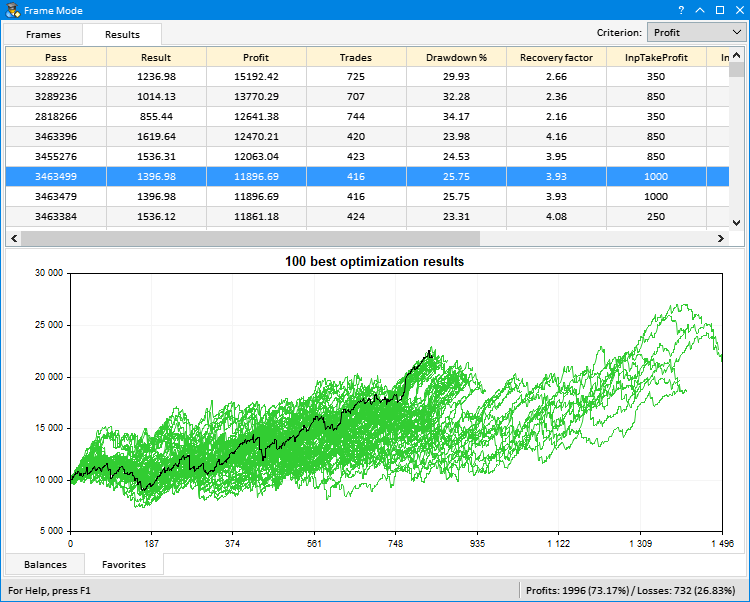Fig. 5. Highlighting the table rows from keyboard

### Conclusion

The article has demonstrated yet another case where the EasyAndFast GUI library can be applied. Obviously, the visual component is very important for the analysis of test results. The deep and extensive view of the arrays of these test results may lead to new ideas. Some of them have already been proposed by the participants of the MQL community.

For example, you can store complete test reports, not just statistical indicators and balance data, in the frames array. Another idea discussed at the forum is to use a custom criterion in the selection of results. For example, you can use several drop-down lists or check boxes to form a custom criterion to be calculated using the equation specified in the settings. It is difficult to imagine how all this could be implemented without a GUI.

Ideas suggested by you in the comments to the article can be implemented in one of the following versions. So do not hesitate to offer your options on how to further develop the application to work with optimization results.

Below, you can download the files for testing and detailed study of the code provided in the article.

File name Comment
MacdSampleCFrames.mq5 Modified EA from the standard delivery - MACD Sample
Program.mqh File with the program class
CreateGUI.mqh File implementing methods from the program class in Program.mqh file
Strategy.mqh File with the modified MACD Sample strategy class (multi-symbol version)
FormatString.mqh File with auxiliary functions for strings formatting
FrameGenerator.mqh File with a class for working with optimization results.

Translated from Russian by MetaQuotes Software Corp.
Original article: https://www.mql5.com/ru/articles/4636

Attached files |
MQL5.zip (74.2 KB)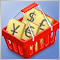Testing currency pair patterns: Practical application and real trading perspectives. Part IV

This article concludes the series devoted to trading currency pair baskets. Here we test the remaining pattern and discuss applying the entire method in real trading. Market entries and exits, searching for patterns and analyzing them, complex use of combined indicators are considered.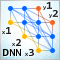Deep Neural Networks (Part VII). Ensemble of neural networks: stacking

We continue to build ensembles. This time, the bagging ensemble created earlier will be supplemented with a trainable combiner — a deep neural network. One neural network combines the 7 best ensemble outputs after pruning. The second one takes all 500 outputs of the ensemble as input, prunes and combines them. The neural networks will be built using the keras/TensorFlow package for Python. The features of the package will be briefly considered. Testing will be performed and the classification quality of bagging and stacking ensembles will be compared.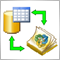Integrating MQL-based Expert Advisors and databases (SQL Server, .NET and C#)

The article describes how to add the ability to work with Microsoft SQL Server database server to MQL5-based Expert Advisors. Import of functions from a DLL is used. The DLL is created using the Microsoft .NET platform and the C# language. The methods used in the article are also suitable for experts written in MQL4, with minor adjustments.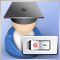Expert Advisor featuring GUI: Adding functionality (part II)

This is the second part of the article showing the development of a multi-symbol signal Expert Advisor for manual trading. We have already created the graphical interface. It is now time to connect it with the program's functionality.